## 2.17Internal energy

The conservation of energy Eq. (2.51 ) introduces the internal energy using the speciﬁc quantity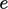, measured in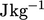in SI units. It represents the total molecular energy consisting of: kinetic energy associated with temperature; potential energy due to particle forces, both within particles as chemical bonds, and between particles, e.g. van der Waals forces.

The energies at diﬀerent scales can be summarised as:

• bulk — kinetic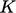due to bulk motion, potential due to forces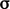and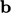;
• molecular — kinetic characterised by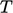, potential due to bonds.

To understand how energy is transferred between these scales, we can derive an equation for internal energy from Eq. (2.51 ), by cancelling terms in mechanical energy formed by taking the inner product of Eq. (2.19 ) with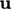.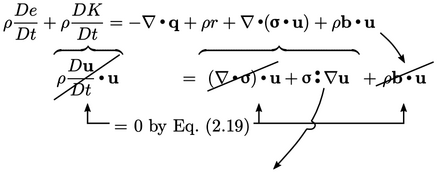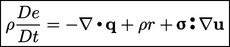(2.56)

The identity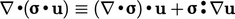is the key element of the analysis. The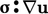term in Eq. (2.56 ) must represent the contribution of mechanical power to the internal energy, i.e. passing from bulk to molecular scale. Conversely, the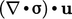term must contribute to mechanical energy.

Substituting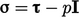from Eq. (2.41 ), Eq. (2.56) becomes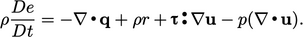(2.57)
The sign of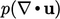depends on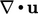, i.e. whether the ﬂuid is expanding or contracting. Since the sign can change, it therefore represents a recoverable contribution to internal energy.

If we substitute the Newtonian model from Eq. (2.41 )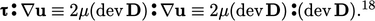(2.58)
The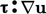term is always positive since all components of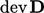are squared. Its contribution to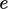is therefore non-recoverable and represents mechanical power that is dissipated as heat. In the majority of CFD analyses, thecontribution is small and can be ignored.

### Double inner product of two tensors

The double inner product of two tensors, denoted by “”, is introduced in Eq. (2.56). It produces a scalar which is evaluated as the sum of the 9 products of the tensor components, eg: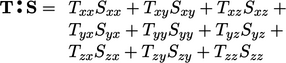(2.59)
For a scalar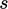,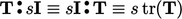.
18This identity relies on the substitution of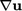by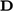which is equivalent due to symmetry of.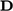can then be replaced by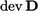since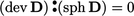.

Notes on CFD: General Principles - 2.17 Internal energy